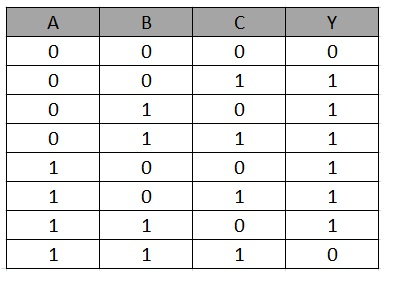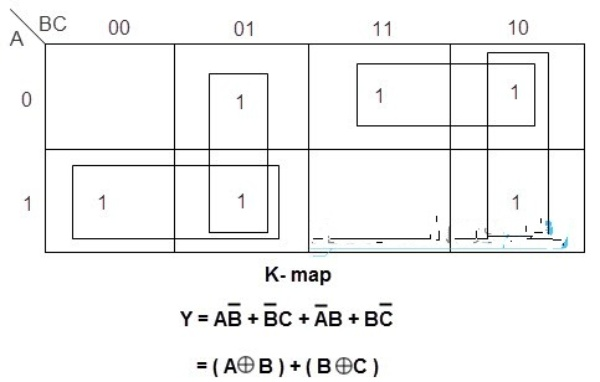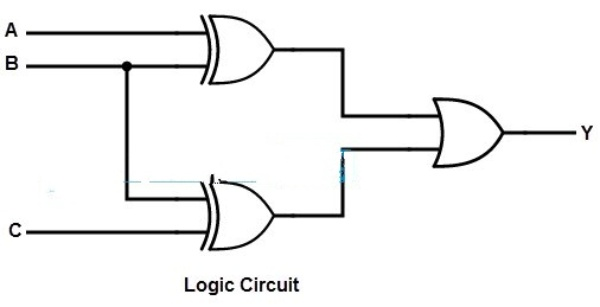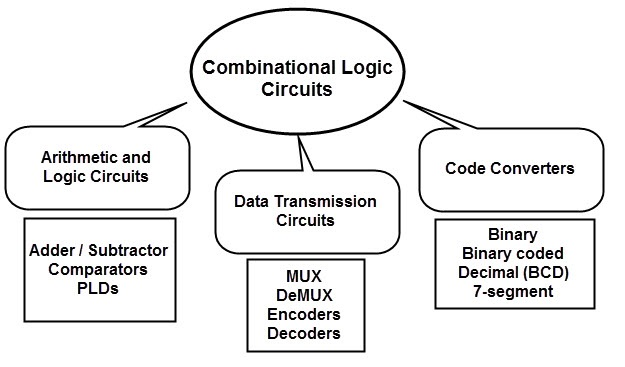### Example of Combinational Logic Circuit

Create a combinational logic circuit with three input variables that outputs logic 1 when one or two of the input variables are logic 1, but not all three.

Solution: Design the logic diagram according to the provided statement using the points listed above. There are three input variables and one output variable in the given statement. Assign the letter symbols A, B, and C to the input variables, and Y to the output. After that, design the truth table as shown below to tabulate the relationship between the input and output variables.Now, using K-map simplification, we can derive the simplified Boolean expression for the above truth table to yield the result Y.By implementing the above Boolean equation we get the logic diagram asClassification of Combinational Logic Circuits

Calculators, digital measurement techniques, computers, digital processing, automatic control of machines, industrial processing, digital communications, and other applications require combinational circuits.

For diverse applications, numerous types of combinational logic circuits are utilised.

Combinational logic circuits are grouped into three types based on the function of the logic circuit: Arithmetic and logic circuits, data transmission circuits, and code converter circuits.1. Arithmetic and Logic Circuits:
• Most computers and calculators have arithmetic operations as one of their primary functions. Logic gates, or simply combinational circuits, carry out these actions by combining many logic gates to provide the desired function. Addition, subtraction, multiplication, and other arithmetic functions are available in combinational circuits.

1. Circuits for Data Transmission:
• Multiplexers and de-multiplexers are the most popular combinational circuits. A multiplexer logic circuit accepts many data inputs and simultaneously passes one of them through the output. These are employed in digital systems for data selection, parallel to series conversion, and data routing.

• A de-multiplexer circuit works in the opposite direction of a multiplexer. It takes a single input and splits it between numerous outputs. These are commonly found in distributors and series to parallel converters.

• Encoders and decoders are two more important data transmission circuits. A decoder logic circuit transforms an n-bit binary input code into 2n output lines. Only one of the potential input combinations is engaged for each output line.

• Data demultiplexing, digital to analogue converters, and digital display applications all use them. As a reverse action of the decoder, an encoder digital circuit converts an active input signal into a coded output signal. These are employed in applications that compress data.

1. Circuits for code converters:
• In some applications, two digital blocks with distinct coding schemes must be interfaced. To convert the information, a conversion circuit is employed in between such circuits. Binary to grey code, grey to binary, BCD to excess-3 code, Excess-3 to BCD code, and seven segment code converter circuits are examples of these converters.

• Types of ADC Circuit Designs | Flash, Successive Approximation Register, Dual Slope

• We live in a world where communication, convenience, and data processing are all digital. As a result, there is an ongoing task of translating data from one domain to another, such as from analogue to digital or vice versa. Analog to Digital Converters (ADC) or Digital to Analog Converters (DAC) are the terms used to describe these data converters (DAC). Let us concentrate on analogue to digital converters in this guide, seeing how a simple ADC works and the various sorts of ADC Circuit Designs.

• This isn't a comprehensive reference to Analog to Digital Converters and the various varieties of ADCs; rather, it's a quick overview of several key ADC circuit types. Analog to Digital Conversion is a vast and fascinating subject in and of itself, and if you're interested, we can create a series of guides for each of the ADC Circuits described below.

Importance of Analog to Digital Conversion

• An ADC (Analog to Digital Converter) is a device that converts analogue signals into digital signals. It is a device or circuit that converts analogue data into digital data (a continuous signal in time with variable magnitude) (discrete data with fixed magnitudes).

• The fundamental advantage of digital data is its ease of storage, as well as the low risk of data corruption caused by analogue signals. As a result, the majority of computing has shifted to the digital domain.

• Digital data has the added benefit of being accurate (depending on the ADC process) and simple to manipulate at high rates.

• Our World, on the other hand, is analogue. Temperature, pressure, light, gas, and other physical characteristics in the real world are continuous analogue signals. As a result, we must utilise data converters like ADCs to transform these analogue signals into digital values so that our digital devices can process, store, analyse, and compute the data quickly.

• When we want to measure, analyse, or process analogue data, we normally convert it to digital data using one of the methods listed below, then process the digital data before converting it back to analogue data using Digital to Analog Converters (DAC).

## Anatomy of a Typical ADC

• ADCs are clearly an important aspect of current signal processing. Let's take a quick look at how a conventional ADC process works before moving on to different sorts of ADC Circuit Designs.

• The initial stage in ADC is to transform physical values like temperature, pressure, and humidity (or any other analogue quantity) into an electrical signal (either current or voltage). Sensors, which are devices that detect changes in physical quantities and output an equivalent electrical signal, are used to do this (usually voltage).

• After that, we must condition the sensor's output. This is because the sensor's output (typically voltage) is frequently in millivolts (very low magnitude). We convert this small electrical signal into a comparatively large signal by using signal conditioners such as amplifiers and filters (usually amplified by a factor of 100 or 1000).

• The Sample and Hold Circuit is the heart of the analogue to digital conversion. We need a device to hold the analogue signal while we process it because it is always changing. The sample and hold circuit will sample the analogue signal and hold it for a period of time determined by the timing circuit (clock circuit).

• The data from the sample and hold block is translated into a digital code depending on the type of ADC Circuit we employ.

• Because of the continuous nature of the analogue signal, there is always a compromise between conversion speed and sample rate when using ADCs.

• Analog to Digital Converter Designs come in a variety of shapes and sizes.

• The following are some of the most prevalent ADC circuit designs:

• ADC with a ramp or a counter

• We'll go over each of these ADC Circuits briefly.

• The Counter ADC is the least expensive but also the slowest of all ADC Circuit designs. Tracking ADC is another name for it. A Voltage Comparator, a Counter, and a DAC are the primary components of this ADC Circuit (Digital to Analog Converter). That's correct. The design of this ADC includes a DAC.

• The main analogue signal is fed into one of the voltage comparator's inputs. The analogue output of the DAC is connected to the comparator's other input. The binary output of the counter is used as the DAC's input.

• The control circuit starts the conversion process by setting the counter to 0 and progressively increasing the count based on the clock signal. The DAC will provide an appropriate analogue signal for the counter data, and the control circuit will halt the counter as soon as the DAC output voltage exceeds the main analogue input voltage.

• The final digital data is the value of the counter when the DAC exceeds the main analogue input.

• For a long time, the Successive Approximation Register (SAR) type ADC has been a popular implementation. The block diagram of the SAR ADC is similar to that of the Counter ADC, with the exception that instead of the main Counter, we have a Register and Latch Circuit.

• The SAR ADC compares the analogue input to the DAC output in a series of comparisons, as the name implies. A binary-weighted code from the consecutive approximation registers is fed into the DAC.

• The MSB of the DAC's input is set to 1 at first, with all other bits set to 0s. The output of the DAC corresponding to this input (which is actually half of its maximum range) is now compared to the input analogue voltage.

• When the analogue input voltage exceeds the DAC's output, the comparator's output becomes HIGH (i.e., 1). As a result, the control logic keeps the MSB at 1 while also setting the bit adjacent to it to 1.

• The current bit is set to 0 and the next significant bit is set to 1 if the DAC output is larger than the analogue input voltage. This operation is repeated until the result is close to the analogue input value.

• Next, we have the Dual Slope ADC, which is a high-speed and high-resolution converter. Because it uses an Integrator circuit in the ADC design, it's also known as Integrating ADC.

• The main analogue input voltage and a reference voltage are both switchable inputs on the integrator. This type of ADC is called as Dual Slope ADC because the integrator provides two separate ramp signals (according to the two inputs).

• The integrator's main analogue input is first set, and the integrator's output is then charged to this value. The integrator's input is set to the reference voltage (which has the opposite polarity as the input analogue signal) after a particular amount of time (specific clock cycles), and the integrator's output charges down to this value.

• The counter starts counting during both of these ramps and stops when the integrator output becomes 0. The digital code that corresponds to the input analogue voltage is the counter's value.